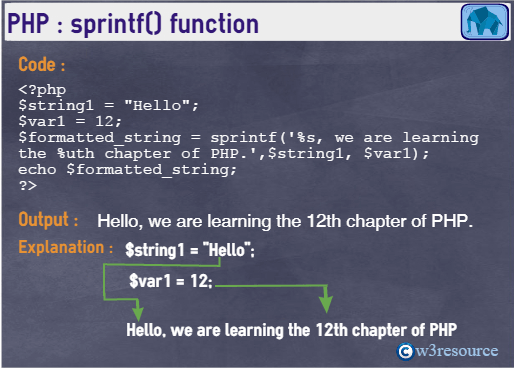# PHP: sprintf() function

## Description

The sprintf() function creates a formatted string from one or more arguments.

Version:

(PHP 4 and above)

Syntax:

`sprintf(format_string ,arg1, arg2, arg3....)`

Parameters:

Name Description Required /
Optional
Type
format Each conversion specification starts with a single percent sign (%) and ends with the following conversion characters.
% - returns a percent sign.
b - the argument is treated as an integer and display it as a binary number.
c - the argument is treated as an integer and display it as a ASCII value.
d - the argument is treated as an integer and display as a signed decimal number.
e - the argument is treated as scientific notation (e.g. 1.2e+2).
E - the argument treated as scientific notation (e.g.1.2E+2).
u - the argument is treated as an integer, and display as an unsigned decimal number.
f- the argument is treated as a float, and display as a floating-point number. (local aware)
F - the argument is treated as a float, and display as a floating-point number (non-locale aware).
g - shorter of %e and %f.
G - shorter of %E and %f.
o- the argument is treated as an integer, and display as an octal number.
s - the argument is treated as string and display as a string.
x - the argument is treated as an integer and display as a hexadecimal number (with lowercase letters).
X - the argument is treated as an integer and display as a hexadecimal number (with uppercase letters).

Optional specification :
Sign specifier : display a sign (+ or -) in front a number. By default a - sign is used in front of a number if it is negative.

Default character is space. An alternate padding character can be specified by prefixing it with a single quote.
Alignment specifier. : - character makes the alignment left-justified. The default is right justified.
Width specifier : An integer number specifies the width of the field.
Precision specifier : The argument specify how many decimal number should be displayed for floating numbers. When using this specifier on a string, it acts as a cutoff point, setting a maximum character limit to the string.
Required String
arg2, arg3... These arguments will added as second %, third % etc. in the formatted string. Optional Mixed

*Mixed : Mixed indicates that a parameter may accept multiple (but not necessarily all) types.

Return value:

A formatted string

Value Type: String.

Pictorial PresentationExample - 1:

``````<?php
\$string1 = "Hello";
\$var1 = 12;
\$formatted_string = sprintf('%s, we are learning the %uth chapter of PHP.',\$string1, \$var1);
echo \$formatted_string;
?>``````

Output:

`Hello, we are learning the 12th chapter of PHP.`

View the example1 in the browser

Example - 2:

``````<?php
\$var1 = 5008;
\$var2 = -5008;
echo sprintf("Binary format  of   \$var1 = '%b'", \$var1).'<br>';
echo sprintf("Hexadecimal format (lower case)  of   \$var1 = '%x'", \$var1).'<br>';
echo sprintf("Hexadecimal format (upper case)  of   \$var1 = '%X'", \$var1).'<br>';
echo sprintf("Octal format of   \$var1 = '%o'", \$var1).'<br>';
echo sprintf("Scientific notation format of   \$var1 = '%e'", \$var1).'<br>';
echo sprintf("Unsigned integer representation of a positive integer format of \$var1 = '%u'", \$var1).'<br>';
echo sprintf("Unsigned integer representation of a negative integer format of \$var1 = '%u'", \$var1).'<br>';
echo sprintf("Floating point representation of a negative integer format of \$var1 = '%f'", \$var1).'<br>';
echo sprintf("Floating point representation of a negative integer format of \$var1 = '%f'", \$var1).'<br>';
echo sprintf("Floating point representation of a negative integer format of \$var1 = '%f'", \$var1).'<br>';
echo sprintf("Sign specifier on a positive integer format of \$var1 = '%+d'", \$var1).'<br>';
echo sprintf("Sign specifier on a positive integer format of \$var2 = '%+d'", \$var2).'<br>';
?>``````

Output:

```Binary format  of   5008 = '1001110010000'
Hexadecimal format (lower   case)  of   5008 = '1390'
Hexadecimal format (upper case)  of   5008 =   '1390'
Octal format of   5008 = '11620'
Scientific notation   format of   5008 = '5.008000e+3'
Unsigned integer representation of a positive integer format of 5008 = '5008'
Unsigned integer representation of a negative integer format of 5008 = '5008'
Floating   point representation of a negative integer format of 5008 =   '5008.000000'
Floating point representation of a negative integer   format of 5008 = '5008.000000'
Floating point representation of a negative integer format of 5008 = '5008.000000'
Sign specifier on a positive integer format of 5008 = '+5008'
Sign specifier on a positive integer format of -5008 = '-5008'```

View the example2 in the browser

PHP Function Reference

Previous: similar_text
Next: sscanf

﻿

## PHP: Tips of the Day

PHP: Pass a PHP string to a JavaScript variable (and escape newlines)

```<script>
var myvar = <?php echo json_encode(\$myVarValue); ?>;
</script>
```

Using json_encode() requires:

• PHP 5.2.0 or greater
• \$myVarValue encoded as UTF-8 (or US-ASCII, of course)

Since UTF-8 supports full Unicode, it should be safe to convert on the fly.

Note that because json_encode escapes forward slashes, even a string that contains </script> will be escaped safely for printing with a script block.

Ref : https://bit.ly/34f0Dym